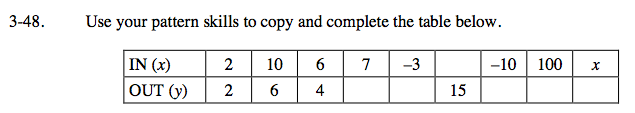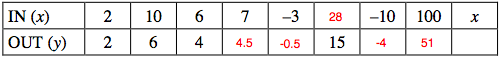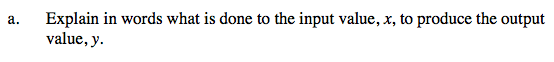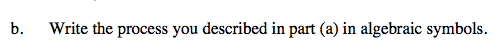### Home > CAAC > Chapter 3 > Lesson 3.1.5 > Problem3-48

3-48.Use the equation y = mx + b to complete the pattern. (m = growth, b = starting point)Describe the equation you found from the values in the table.

Divide x by 2 and then add 1.